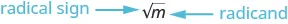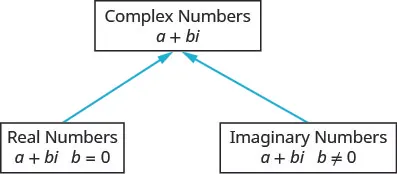Intermediate Algebra 2e

# Key Concepts

### Key Concepts

#### 8.1Simplify Expressions with Roots

• Square Root Notation
• $mm$ is read ‘the square root of m
• If n2 = m, then $n=m,n=m,$ for $n≥0.n≥0.$• The square root of m, $m,m,$ is a positive number whose square is m.
• nth Root of a Number
• If $bn=a,bn=a,$ then b is an nth root of a.
• The principal nth root of a is written $an.an.$
• n is called the index of the radical.
• Properties of $anan$
• When n is an even number and
• $a≥0,a≥0,$ then $anan$ is a real number
• $a<0,a<0,$ then $anan$ is not a real number
• When n is an odd number, $anan$ is a real number for all values of a.
• Simplifying Odd and Even Roots
• For any integer $n≥2,n≥2,$
• when n is odd $ann=aann=a$
• when n is even $ann=|a|ann=|a|$
• We must use the absolute value signs when we take an even root of an expression with a variable in the radical.

• For real numbers a, m and $n≥2n≥2$
$anan$ is considered simplified if a has no factors of $mnmn$
• Product Property of nth Roots
• For any real numbers, $anan$ and $bn,bn,$ and for any integer $n≥2n≥2$
$abn=an·bnabn=an·bn$ and $an·bn=abnan·bn=abn$
• How to simplify a radical expression using the Product Property
1. Step 1. Find the largest factor in the radicand that is a perfect power of the index.
Rewrite the radicand as a product of two factors, using that factor.
2. Step 2. Use the product rule to rewrite the radical as the product of two radicals.
3. Step 3. Simplify the root of the perfect power.
• Quotient Property of Radical Expressions
• If $anan$ and $bnbn$ are real numbers, $b≠0,b≠0,$ and for any integer $n≥2n≥2$ then,
$abn=anbnabn=anbn$ and $anbn=abnanbn=abn$
• How to simplify a radical expression using the Quotient Property.
1. Step 1. Simplify the fraction in the radicand, if possible.
2. Step 2. Use the Quotient Property to rewrite the radical as the quotient of two radicals.
3. Step 3. Simplify the radicals in the numerator and the denominator.

#### 8.3Simplify Rational Exponents

• Rational Exponent $a1na1n$
• If $anan$ is a real number and $n≥2,n≥2,$ then $a1n=an.a1n=an.$
• Rational Exponent $amnamn$
• For any positive integers m and n,
$amn=(an)mamn=(an)m$ and $amn=amnamn=amn$
• Properties of Exponents
• If a, b are real numbers and m, n are rational numbers, then
• Product Property $am·an=am+nam·an=am+n$
• Power Property $(am)n=am·n(am)n=am·n$
• Product to a Power $(ab)m=ambm(ab)m=ambm$
• Quotient Property $aman=am−n,a≠0aman=am−n,a≠0$
• Zero Exponent Definition $a0=1,a0=1,$ $a≠0a≠0$
• Quotient to a Power Property $(ab)m=ambm,b≠0(ab)m=ambm,b≠0$
• Negative Exponent Property $a−n=1an,a≠0a−n=1an,a≠0$

• Product Property of Roots
• For any real numbers, $anan$ and $bn,bn,$ and for any integer $n≥2n≥2$
$abn=an·bnabn=an·bn$ and $an·bn=abnan·bn=abn$
• Special Products
$Binomial SquaresProduct of Conjugates(a+b)2=a2+2ab+b2(a+b)(a−b)=a2−b2(a−b)2=a2−2ab+b2Binomial SquaresProduct of Conjugates(a+b)2=a2+2ab+b2(a+b)(a−b)=a2−b2(a−b)2=a2−2ab+b2$

• Quotient Property of Radical Expressions
• If $anan$ and $bnbn$ are real numbers, $b≠0,b≠0,$ and for any integer $n≥2n≥2$ then,
$abn=anbnabn=anbn$ and $anbn=abnanbn=abn$
• A radical expression is considered simplified if there are:
• no factors in the radicand that have perfect powers of the index
• no fractions in the radicand
• no radicals in the denominator of a fraction

• Binomial Squares
$(a+b)2=a2+2ab+b2(a−b)2=a2−2ab+b2(a+b)2=a2+2ab+b2(a−b)2=a2−2ab+b2$
1. Step 1. Isolate one of the radical terms on one side of the equation.
2. Step 2. Raise both sides of the equation to the power of the index.
3. Step 3. Are there any more radicals?
If yes, repeat Step 1 and Step 2 again.
If no, solve the new equation.
4. Step 4. Check the answer in the original equation.
• Problem Solving Strategy for Applications with Formulas
1. Step 1. Read the problem and make sure all the words and ideas are understood. When appropriate, draw a figure and label it with the given information.
2. Step 2. Identify what we are looking for.
3. Step 3. Name what we are looking for by choosing a variable to represent it.
4. Step 4. Translate into an equation by writing the appropriate formula or model for the situation. Substitute in the given information.
5. Step 5. Solve the equation using good algebra techniques.
6. Step 6. Check the answer in the problem and make sure it makes sense.
7. Step 7. Answer the question with a complete sentence.
• Falling Objects
• On Earth, if an object is dropped from a height of h feet, the time in seconds it will take to reach the ground is found by using the formula $t=h4.t=h4.$
• Skid Marks and Speed of a Car
• If the length of the skid marks is d feet, then the speed, s, of the car before the brakes were applied can be found by using the formula $s=24d.s=24d.$

• Properties of $anan$
• When n is an even number and:
$a≥0,a≥0,$ then $anan$ is a real number.
$a<0,a<0,$ then $anan$ is not a real number.
• When n is an odd number, $anan$ is a real number for all values of a.
• Domain of a Radical Function
• When the index of the radical is even, the radicand must be greater than or equal to zero.
• When the index of the radical is odd, the radicand can be any real number.

#### 8.8Use the Complex Number System

• Square Root of a Negative Number
• If b is a positive real number, then $−b=bi−b=bi$
 $a+bia+bi$ $b=0b=0$ $a+0·iaa+0·ia$ Real number $b≠0b≠0$ $a+bia+bi$ Imaginary number $a=0a=0$ $0+bibi0+bibi$ Pure imaginary number
Table 8.1
• A complex number is in standard form when written as a + bi, where a, b are real numbers.• Product of Complex Conjugates
• If a, b are real numbers, then
$(a−bi)(a+bi)=a2+b2(a−bi)(a+bi)=a2+b2$
• How to Divide Complex Numbers
1. Step 1. Write both the numerator and denominator in standard form.
2. Step 2. Multiply the numerator and denominator by the complex conjugate of the denominator.
3. Step 3. Simplify and write the result in standard form.
Order a print copy

As an Amazon Associate we earn from qualifying purchases.